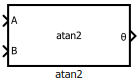Main Content

# atan2

Compute four-quadrant arctangent

• Library:
• Motor Control Blockset / Controls / Math Transforms

•## Description

The atan2 block performs the four-quadrant arctangent on two real numbers.

### Equations

This equation describes how the block computes the four-quadrant arctangent (`θ`).

where:

## Ports

### Input

expand all

Real number on the y-axis that you provide as input to the block.

Data Types: `single` | `double` | `fixed point`

Real number on the x-axis that you provide as input to the block.

Data Types: `single` | `double` | `fixed point`

### Output

expand all

Angle represented by arctangent. This is the angle made by a vector from the origin to a specified point (x,y) with the positive x-axis.

Data Types: `single` | `double` | `fixed point`

The following figure shows the representation of input values A, B, and arctangent on the x-y coordinate plane.## Parameters

expand all

Unit of the output values.

## Extended Capabilities

### Fixed-Point ConversionDesign and simulate fixed-point systems using Fixed-Point Designer™.

Introduced in R2020a

Get ebook